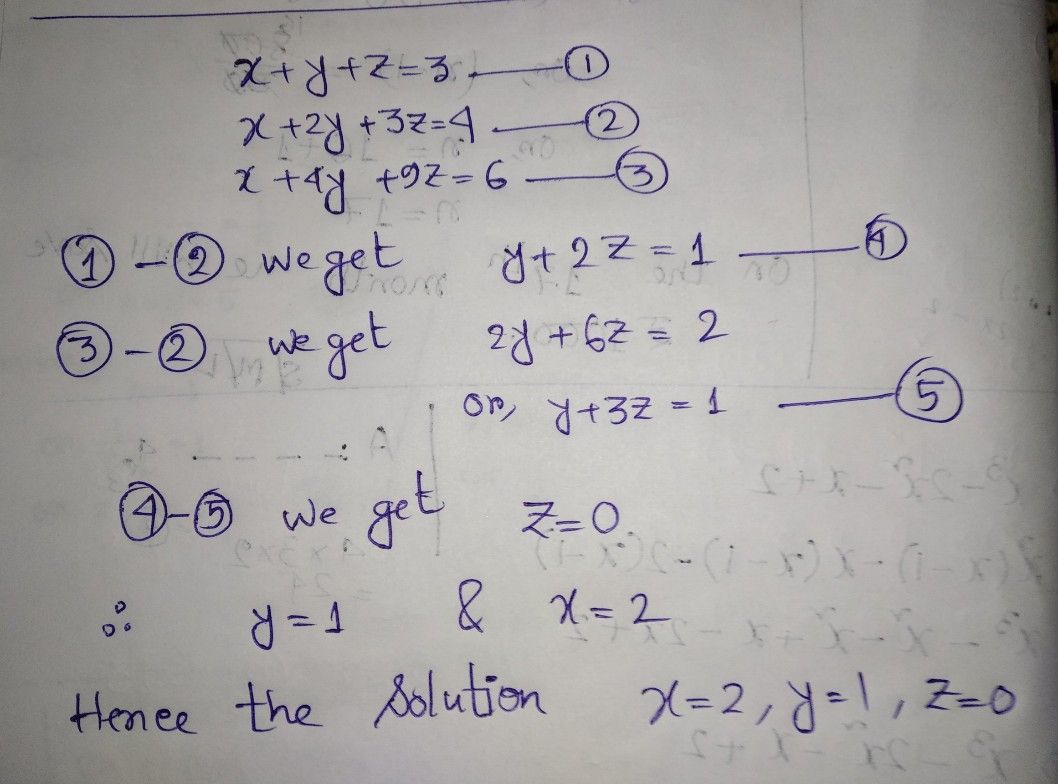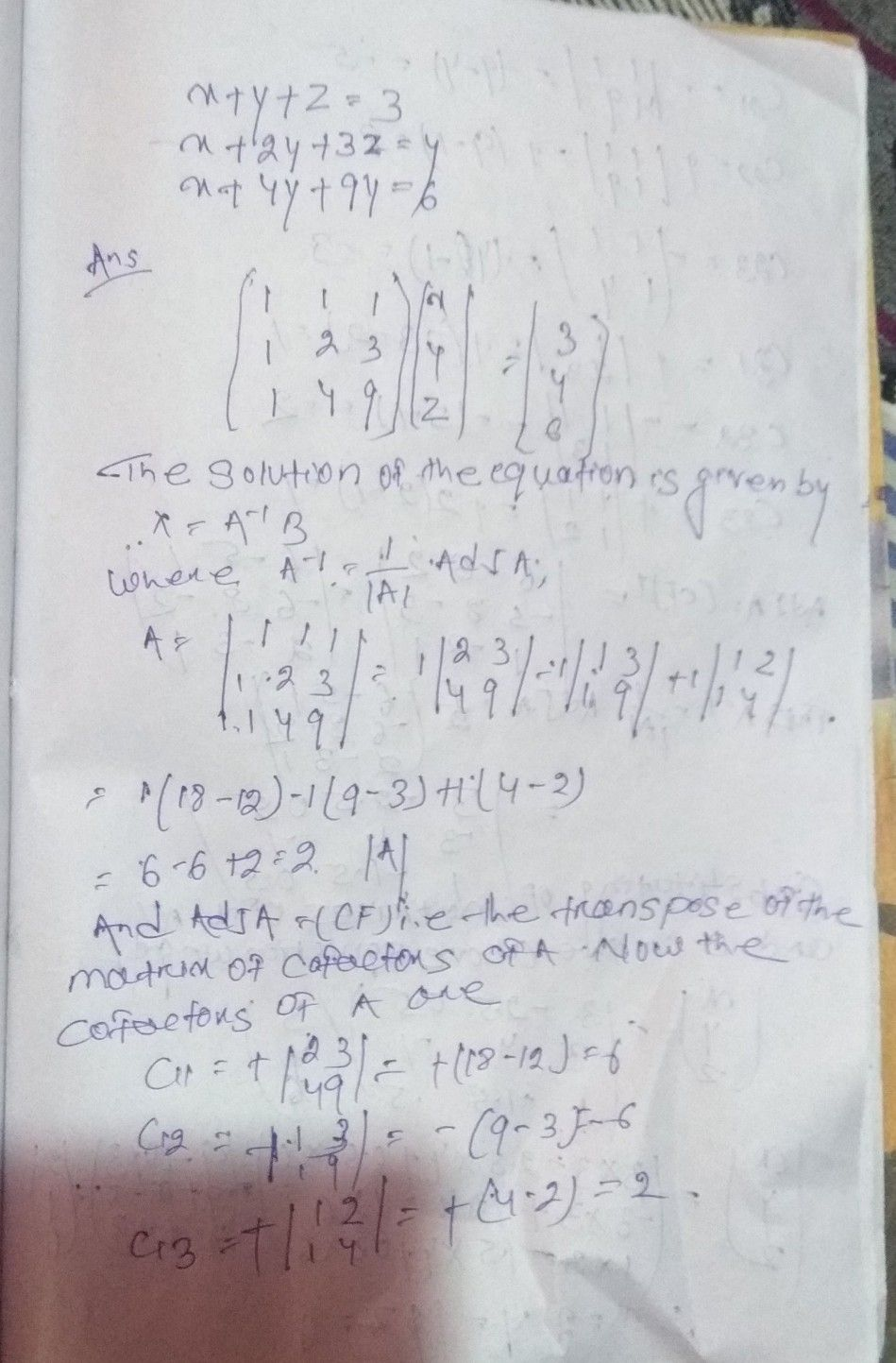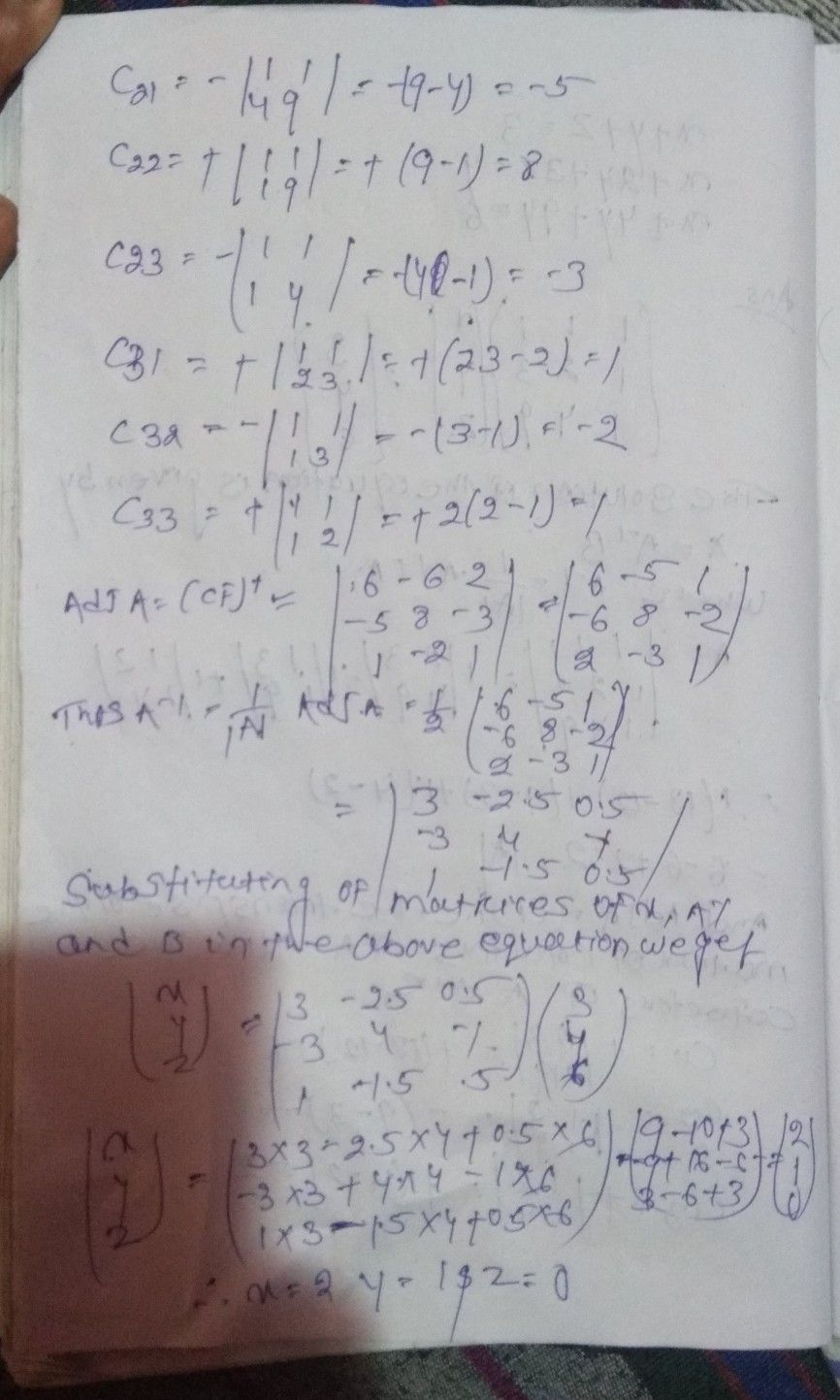Symbol
Problem$x+y+z=3$ $x+2y+3z=4$ $x+4y+9z=6$ $m$ $4^{°}$ $41$
Other
SolutionQanda teacher - Sayan RayStudent
I don't understand. What you have doneQanda teacher - Sayan Ray
Which stepStudent
4th to lastQanda teacher - Sayan Ray
Have you understand 4th and 5th equationStudent
NoRight AnswerQanda teacher - Sayan Ray
My answer is also right and my process is much less than this matrix method